# Apply a function to each row or column in Dataframe using pandas.apply()

• Difficulty Level : Basic
• Last Updated : 19 Jul, 2021

There are different ways to apply a function to each row or column in DataFrame. We will learn about various ways in this post. Let’s create a small dataframe first and see that.

Attention geek! Strengthen your foundations with the Python Programming Foundation Course and learn the basics.

To begin with, your interview preparations Enhance your Data Structures concepts with the Python DS Course. And to begin with your Machine Learning Journey, join the Machine Learning - Basic Level Course

## Python3

 `# import pandas and numpy library``import` `pandas as pd``import` `numpy as np`` ` `# list of tuples``matrix ``=` `[(``1``,``2``,``3``,``4``),``          ``(``5``,``6``,``7``,``8``,),``          ``(``9``,``10``,``11``,``12``),``          ``(``13``,``14``,``15``,``16``)``         ``]`` ` `# Create a Dataframe object``df ``=` `pd.DataFrame(matrix, columns ``=` `list``(``'abcd'``))`` ` `# Output``df`

Output :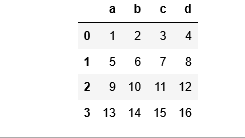Method 1: Applying lambda function to each row/column.
Example 1: For Column

## Python3

 `# import pandas and numpy library``import` `pandas as pd``import` `numpy as np` `# list of tuples``matrix ``=` `[(``1``,``2``,``3``,``4``),``          ``(``5``,``6``,``7``,``8``,),``          ``(``9``,``10``,``11``,``12``),``          ``(``13``,``14``,``15``,``16``)``         ``]` `# Create a Dataframe object``df ``=` `pd.DataFrame(matrix, columns ``=` `list``(``'abcd'``))` `# Applying a lambda function to each``# column which will add 10 to the value``new_df ``=` `df.``apply``(``lambda` `x : x ``+` `10``)` `# Output``new_df`

Output :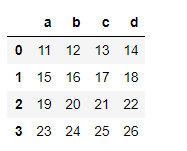Example 2: For Row

## Python3

 `# import pandas and numpy library``import` `pandas as pd``import` `numpy as np` `# list of tuples``matrix ``=` `[(``1``,``2``,``3``,``4``),``          ``(``5``,``6``,``7``,``8``,),``          ``(``9``,``10``,``11``,``12``),``          ``(``13``,``14``,``15``,``16``)``         ``]` `# Creating a Dataframe object``df ``=` `pd.DataFrame(matrix, columns ``=` `list``(``'abcd'``))` `# Applying a lambda function to each``# row which will add 5 to the value``new_df ``=` `df.``apply``(``lambda` `x: x ``+` `5``, axis ``=` `1``)` `# Output``new_df`

Output :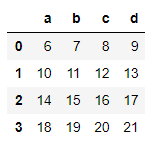Method 2: Applying user defined function to each row/column
Example 1: For Column

## Python3

 `# function to returns x*x``def` `squareData(x):``    ``return` `x ``*` `x` `# import pandas and numpy packages``import` `pandas as pd``import` `numpy as np` `# list of tuples``matrix ``=` `[(``1``,``2``,``3``,``4``),``          ``(``5``,``6``,``7``,``8``,),``          ``(``9``,``10``,``11``,``12``),``          ``(``13``,``14``,``15``,``16``)``         ``]` `# Creating a Dataframe object``df ``=` `pd.DataFrame(matrix, columns ``=` `list``(``'abcd'``))` `# Applying a user defined function to``# each column that will square the given``# value``new_df ``=` `df.``apply``(squareData)` `# Output``new_df`

Output :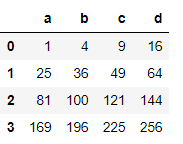Example 2: For Row

## Python3

 `# function to returns x*X``def` `squareData(x):``    ``return` `x ``*` `x` `# import pandas and numpy library``import` `pandas as pd``import` `numpy as np` `# List of tuples``matrix ``=` `[(``1``,``2``,``3``,``4``),``          ``(``5``,``6``,``7``,``8``,),``          ``(``9``,``10``,``11``,``12``),``          ``(``13``,``14``,``15``,``16``)``         ``]` `# Creating a Dataframe object``df ``=` `pd.DataFrame(matrix, columns ``=` `list``(``'abcd'``))` `# Applying a user defined function``# to each row that will square the given value``new_df ``=` `df.``apply``(squareData, axis ``=` `1``)` `# Output``new_df`

Output :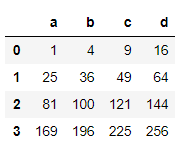In the above examples, we saw how a user defined function is applied to each row and column. We can also apply user defined functions which take two arguments.

Example 1: For Column

## Python3

 `# function to returns x+y``def` `addData(x, y):``    ``return` `x ``+` `y` `# import pandas and numpy library``import` `pandas as pd``import` `numpy as np` `# list of tuples``matrix ``=` `[(``1``,``2``,``3``,``4``),``          ``(``5``,``6``,``7``,``8``,),``          ``(``9``,``10``,``11``,``12``),``          ``(``13``,``14``,``15``,``16``)``         ``]` `# Creating a Dataframe object``df ``=` `pd.DataFrame(matrix, columns ``=` `list``(``'abcd'``))` `# Applying a user defined function to each``# column which will add value in each``# column by given number``new_df ``=` `df.``apply``(addData, args ``=` `[``1``])` `# Output``print``(new_df)`

Output: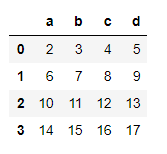Example 2: For Row

## Python3

 `# function to returns x+y``def` `addData(x, y):``    ``return` `x ``+` `y` `# import pandas and numpy library``import` `pandas as pd``import` `numpy as np` `# List of tuples``matrix ``=` `[(``1``,``2``,``3``,``4``),``          ``(``5``,``6``,``7``,``8``,),``          ``(``9``,``10``,``11``,``12``),``          ``(``13``,``14``,``15``,``16``)``         ``]` `# Creating a Dataframe object``df ``=` `pd.DataFrame(matrix, columns ``=` `list``(``'abcd'``))` `# Applying a user defined function to each``# row which will add value in each row by``# given number``new_df ``=` `df.``apply``(addData, axis ``=` `1``,``                    ``args ``=` `[``3``])` `# Output``new_df`

Output :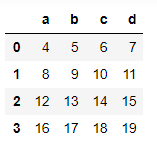Method 3: Applying numpy function to each row/column
Example 1: For Column

## Python3

 `# import pandas and numpy library``import` `pandas as pd``import` `numpy as np` `# list of tuples``matrix ``=` `[(``1``,``2``,``3``,``4``),``          ``(``5``,``6``,``7``,``8``,),``          ``(``9``,``10``,``11``,``12``),``          ``(``13``,``14``,``15``,``16``)``         ``]` `# Creating a dataframe object``df ``=` `pd.DataFrame(matrix, columns ``=` `list``(``'abcd'``))` `# Applying a numpy function to each``# column by squaring each value``new_df ``=` `df.``apply``(np.square)` `# Output``new_df`

Output :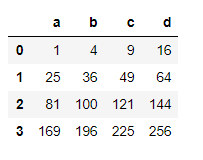Example 2: For Row

## Python3

 `# import pandas and numpy library``import` `pandas as pd``import` `numpy as np` `# List of tuples``matrix ``=` `[(``1``,``2``,``3``,``4``),``          ``(``5``,``6``,``7``,``8``,),``          ``(``9``,``10``,``11``,``12``),``          ``(``13``,``14``,``15``,``16``)``         ``]` `# Creating a dataframe object``df ``=` `pd.DataFrame(matrix, columns ``=` `list``(``'abcd'``))` `# Apply a numpy function to each row``# to find square root of each value``new_df ``=` `df.``apply``(np.sqrt, axis ``=` `1``)` `# Output``new_df`

Output :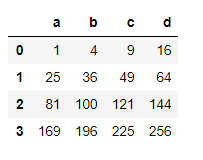Method 4: Applying a Reducing function to each row/column
A Reducing function will take row or column as series and returns either a series of same size as that of input row/column or it will return a single variable depending upon the function we use.

Example 1: For Column

## Python3

 `# import pandas and numpy library``import` `pandas as pd``import` `numpy as np` `# List of tuples``matrix ``=` `[(``1``,``2``,``3``,``4``),``          ``(``5``,``6``,``7``,``8``,),``          ``(``9``,``10``,``11``,``12``),``          ``(``13``,``14``,``15``,``16``)``         ``]` `# Creating a Dataframe object``df ``=` `pd.DataFrame(matrix, columns ``=` `list``(``'abcd'``))` `# Applying a numpy function to get the sum``# of all values in each column``new_df ``=` `df.``apply``(np.``sum``)` `# Output``new_df`

Output :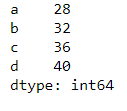Example 2: For Row

## Python3

 `# import pandas and numpy library``import` `pandas as pd``import` `numpy as np` `# List of tuples``matrix ``=` `[(``1``,``2``,``3``,``4``),``          ``(``5``,``6``,``7``,``8``,),``          ``(``9``,``10``,``11``,``12``),``          ``(``13``,``14``,``15``,``16``)``         ``]` `# Creating a Dataframe object``df ``=` `pd.DataFrame(matrix, columns ``=` `list``(``'abcd'``))` `# Applying a numpy function to get t``# he sum of all values in each row``new_df ``=` `df.``apply``(np.``sum``, axis ``=` `1``)` `# Output``new_df`

Output :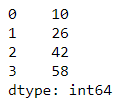My Personal Notes arrow_drop_up